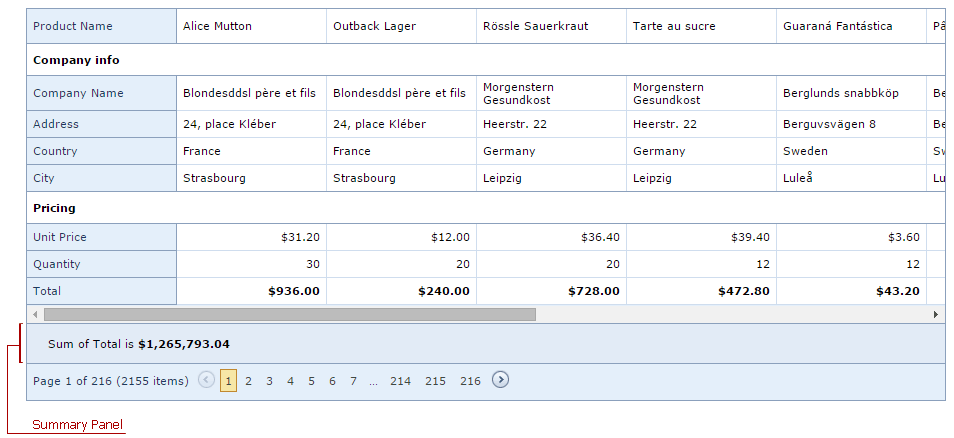# Data Summaries (Vertical Grid)

ASPxVerticalGrid allows you to display summaries, i.e., brief information about individual rows. For example, you can display the number of records.

ASPxVerticalGrid supports total summaries. A total summary is the value of an aggregate function calculated over all the records; this total summary is displayed in the summary panel.A summary is an ASPxVerticalGridSummaryItem object. To work properly, the following two properties must be specified.

``````<dx:ASPxVerticalGridSummaryItem FieldName="Total" SummaryType="Sum"/>
``````

Note

In server mode, a summary cannot be calculated for unbound rows. In this mode, values are calculated using events (see ASPxVerticalGrid.CustomUnboundRowData). Only rows with unbound expressions (see VerticalGridDataRow.UnboundExpression) support summary calculation.